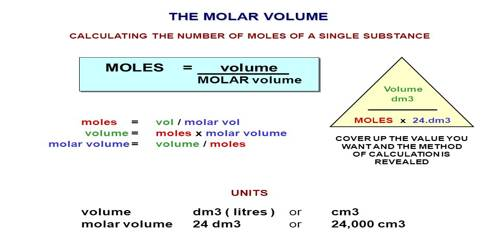# Molar Volume

Molar Volume

The molar volume of any substance is the volume occupied by one mole of the substance. This quantity is easily determined by dividing the molecular mass by the density of the compound. According to Avogadro’s hypothesis, the molar volume of all gases at S. T. P. is 22,414 mL. As Avogadro’s hypothesis is not strictly applicable to all gases slight variations from this value are likely to be observed.

The molar volumes of all liquids, however, show marked addictivity when measured at their respective boiling points wider atmospheric pressure. These observations led Kopp (1842) to state that the molar volume of a liquid at its boiling point is equal to the sum of the atomic volume of its constituent atoms. This is known as Kopp’s law. Kopp’s measurements showed that the molar volumes of two members of a homologous series of organic liquids differ by about 22 mL, for each CH2 group Kopp calculated the volume equivalent of each element by a simple arithmetic means. For example, in a hydrocarbon of the formula CnH2n+2 there are ‘n’ numbers of CH2 groups and two hydrogen atoms. Subtraction of n x 22 mL from the molar volume a liquid hydrocarbon gives the volume equivalent of 2 H atoms. The mean value of the difference comes to 11.0 mL so that the volume equivalent of each hydrogen atom is 5.5 mL. This leaves the volume equivalent of carbon as 11.0 mL. From these values and molar volumes, the volume equivalents of many atoms were calculated. The values of volume equivalents were taken as atomic volumes by Kopp.This law was found to be only approximately correct as deviations are numerous. This is particularly obvious in the case of isomers which, according to Kopp’s law, should have the same molar volume. In a large number of such cases, the difference between the molar volumes of isomers was marked. This may be attributed to structural effects on the molar volume. In spite of its approximate nature, the Lab has been useful in estimating molar volume.